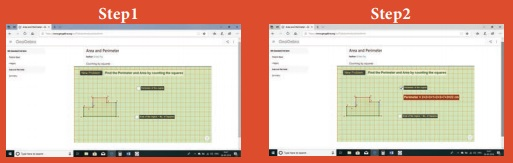Home | | Maths 6th Std | Expressing the Area in Square Units

# Expressing the Area in Square Units

Consider a square of side 1 cm. Therefore, its area is 1 sq. cm. Divide one of its sides into 10 equal parts. One such part is equal to 1 mm. We know that 1 cm = 10 mm.

Expressing the Area in Square Units

Consider a square of side 1 cm. Therefore, its area is 1 sq. cm (1 cm2). Divide one of its sides into 10 equal parts. One such part is equal to 1 mm. We know that 1 cm = 10 mm. That is a square of side 1 cm is made up of 100 small squares with 1 mm square area each. Therefore, the side of this square is 10 mm and the area of this square = side × side =10 mm × 10 mm = 100 sq. mm (100 mm2). Therefore, the area of a square with 1 cm side is 1 cm2 = 100 mm2.

Similarly, the other conversions can also be done. For example,

i) 1 cm2 = 10 mm × 10 mm

= 100 mm2

ii) 1 m2 = 100 cm x 100 cm

= 10,000 cm2

iii) 1 km2 = 1000 m x 1000 m

= 10,00,000 m2Example 15

Fill in the blanks.

i) 2 cm2 = _____ mm2

ii) 18 m2 = _____ cm2

iii) 5 km2 = _____ m2

Solution

i) 2 cm2 = 2 × 100 = 200 mm2

ii) 18 m2 = 18 × 10000 = 1,80,000 cm2

iii) 5 km2 = 5 × 1000000 = 50,00,000 m2

DO YOU KNOW?

1 acre = 4046.86 m2

1 hectare = 10,000 m2

Try these

Fill in the blanks

i) 7 cm2700 mm2

ii) 10 m2100000 cm2

iii) 3 km23000000 m2

ICT CORNER

Perimeter and Area

Expected OutcomeStep – 1

Open the Browser by typing the URL Link given below (or) Scan the QR Code. GeoGebra work sheet named “Perimeter and Area” will open. There is a worksheet under the title Counting by squares.

Step – 2

Click on New Problem and find the perimeter and Area of the shape by counting along the squares. Click on the respective check boxes to check your answer.